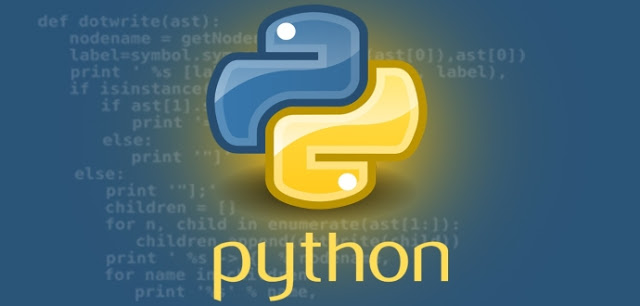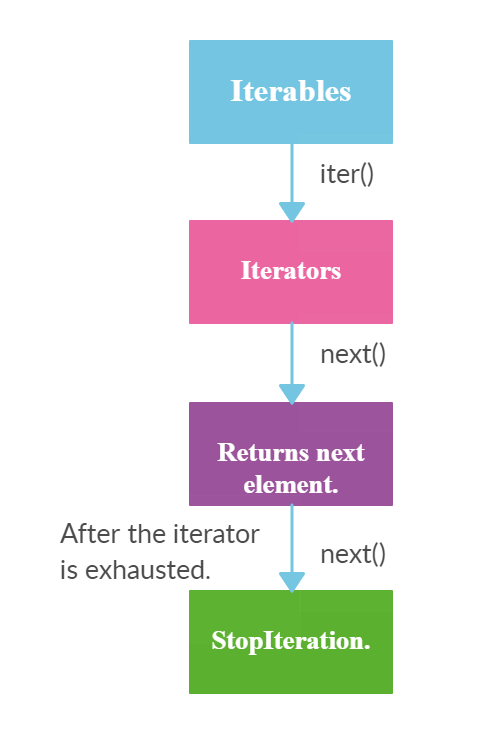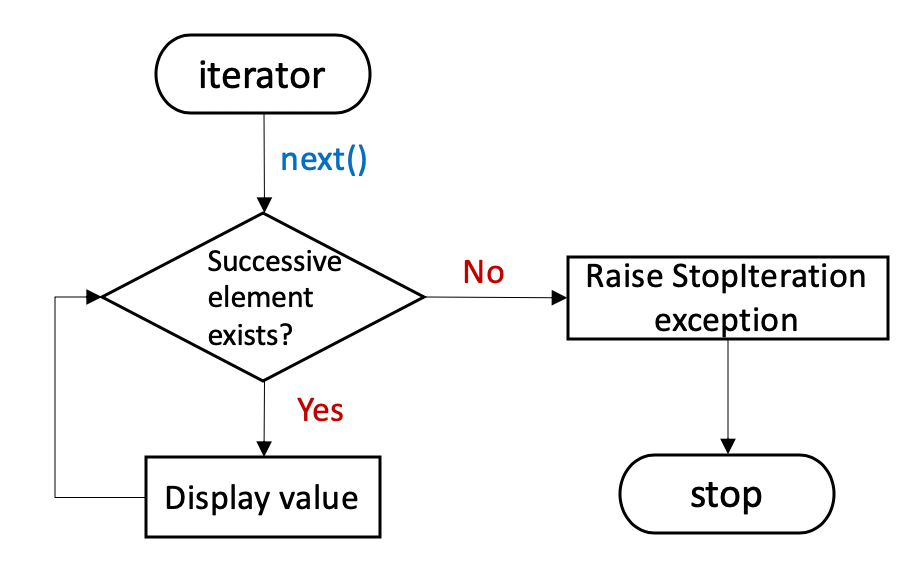CHIRAG GOYAL — Published On July 6, 2021 and Last Modified On August 4th, 2022

This article was published as a part of the Data Science Blogathon

## Introduction

Python is a beautiful Programming Language. Because of its flexibility and incredible functionalities, most people loved this language. While working with Python, I have come across a few functionalities whose usage is not commensurate to the number of complexities they simplify. Sometimes, these are also known as “Hidden Gems” in Python. These hidden things are not known by a lot of people but they become super useful when anyone wants to work in the domain of Analytics and Data Science.

In this category, Python Iterators and Iterables can be fitted. Their potential is immense!Image Source:

One of the most important concepts in Python is Iteration. The term Iteration not comes alone but comes up with two other terms that pertain to iteration are iterators and iterables. Also, the understanding of iterables and iterators in Python is very important. But these python concepts are a little bit tricky to understand. In this article, I will try to give a comprehensive explanation of iterables and iterators. We will understand these things starting from what they are, how they work, and also see how to use them properly.

So let’s deep dive into the article and explore the world of Python Iterators and Iterables.

To follow this article properly, I assume you are familiar with the basics of Python. If not, I recommend the below popular course given by Analytics Vidhya to get started with the Python Basics:

Python for Data Science

• What is Iteration?
• What are Iterables?
• What are Python Iterators?
• Why should you use iterators?
• What is the relationship between Iterators and Iterables?
• What is the difference between Iterators and Iterables?
• What are the Limits of Iterator?

## What is Iteration?

One of the most essential principles of software development is that Don’t Repeat Yourself, which is elaborated in “The Pragmatic Programmer” Book in the following way:

“Every piece of knowledge must have a single, unambiguous, authoritative representation within a system.” — Andy Hunt and Dave Thomas

One specific application of the above-mentioned principle in modern programming is the use of Iteration that involves going over a list of items, on which we performed the defined operation. One of the most basic forms of iteration is a for loop.

Although many other languages such as and use three-expression for-loop, while Python programming language uses a more concise syntax of for-loop.

To understand what exactly iteration means, you have to understand the following points:

• Iteration means ‘repeating steps’ in layman’s language.
• In Programming, Iteration is defined as a repetition of a block of code a specified number of times.
• To achieve the iterations, we can use loops such as for loop, etc.

## What are Iterables?

Iterables are objects that can be iterated in iterations.

To understand what exactly iterables means, you have to understand the following points:

• Iterable is an object which can be looped over or iterated over with the help of a for loop.
• Objects like lists, tuples, sets, dictionaries, strings, etc. are called iterables. In short and simpler terms, iterable is anything that you can loop over.
• In simpler words, iterable is a container that has data or values and we perform an iteration over it to get elements one by one. (Can traverse through all the given values one by one)
• Iterable has an in-built dunder method __iter__.

### How we can check whether an object is Iterable or not?

An object is called an iterable if you can get an iterator out of it.

A simpler way to determine whether an object is iterable is to check if it supports __iter__. How? Here we use the function named dir( ), which returns the list of attributes and methods supported by an object, and by seeing all attributes and methods, we can find all and select required methods from them.

• Calling iter() function on an iterable gives us an iterator.
• Calling the next() function on iterator gives us the next element.
• If the iterator is exhausted(if it has no more elements), calling next() raises the StopIteration exception.Image Source:

For Example,

Python Code:

## What are Python Iterators?

An Iterator is an object representing a stream of data that produces a data value at a time using the __next__() method.

To understand what exactly iterators mean, you have to understand the following points:

• In Python, an iterator is an object which implements the iterator protocol, which means it consists of the methods such as  __iter__() and __next__().
• An iterator is an iterable object with a state so it remembers where it is during iteration. For Example, Generator
• These iterators give or return the data one element at a time.
• It performs the iteration to access the elements of the iterable one by one. As it maintains the internal state of elements, the iterator knows how to get the next value.

### Which in-built methods does iterator have?

Iterator supports in-built dunder methods such as  __iter__ and __next__

Iterators can only move forward using __next__. But remember that, iterators cannot go back or cannot be reset.

• The __next__() method takes no arguments and always returns the next element of the stream.
• If there are no more elements in the stream, __next__() must raise the StopIteration exception.
• Iterators don’t have to be finite. It’s perfectly reasonable to write an iterator that produces an infinite stream of data.

For Example,

```number_iterator = iter([1, 2, 3, 4, 5])
print(type(number_iterator))
print(next(number_iterator))
print(next(number_iterator))
print(next(number_iterator))
print(next(number_iterator))
print(next(number_iterator))
# Once the iterator is exhausted, next() function raise StopIteration.
print(next(number_iterator))```

Output:

Specifically, in the above code, we first created an iterator called number_iterator by passing a list of numbers to the iter() method. When we checked its type with the help of the type() function of Python, we found that it was indeed an iterator, or more specifically a list_iterator. Every time we called the next() method on the number_iterator, the iterator produced an integer for us until the StopIteration exception is raised.

## Why should you use Iterators?

In modern days, we have a lot of data in our hands, and handling this huge amount of data creates problems for everyone who wants to do some sort of analysis with that data.

So, If you’ve ever struggled with handling huge amounts of data, and your machine running out of memory, then you’ll love the concept of Iterators in Python.

Therefore, Rather than putting all the data in the memory in one step, it would be better if we could work with it in bits or some small chunks, dealing with only that data that is required at that moment, right? As a result, this would reduce the load on our computer memory tremendously. And this is what exactly the iterators do!

Therefore, you can use Iterators to save a ton of memory, as Iterators don’t compute their items when they are generated, but only when they are called upon.

## Relationship between Iterators and Iterables

The first thing we have to note is that “Iterators are Iterables”, and if we use the iterator in a for loop, then it will still work.

```number_iterator = iter([1, 2, 3, 4, 5])
for element in number_iterator:
print(element)```

Output:

```1
2
3
4
5```

However, the opposite is not always true. For example, iterables like strings and lists are not iterators such that they don’t have the next() method.

`'Chirag'.__next__()`

Output:

`[1, 2, 3, 4, 5].__next__()`

Output:

Given the above differences between iterables and iterators, they’re used differently in for loops.

Now, as shown in the below example, we use the same iterable (i.e., a list) in two for loops without getting any errors. By contrast, the iterator can be used just once, as completing the first for loop has already made the iterator iterate all elements such that no more elements to be iterated.

```# Use iterables in for-loops for multiple times
number_iterable = [1, 2, 3]
for i in number_iterable:
print(i)
print('-----')
for i in number_iterable:
print(i)
print('-----')
# Use iterators in for-loops for multiple times
number_iterator = iter([1, 2, 3])
for i in number_iterator:
print(i)
print('-----')
for i in number_iterator:
print(i)
# nothing is printed```

Output:

```1
2
3
-----
1
2
3
-----
1
2
3
-----```

## Difference between Iterables and Iterators

Now, in this section we will discuss the difference between Iterables and Iterators:

### Iterables

• Can be iterated using for loop.
• Iterables support iter() function.
• Iterables are not Iterators.

### Iterators

• Can be iterated using for loop.
• Iterators suppports iter() and next() function.
• Iterators are also Iterables.

Let’s understand each point described above with the help of examples:

### 1. Both iterables and iterators can be iterated using for loop.

Let’s understand the above concept with the help of the following example:

```list_1 = [1,2,3,4,5]
list_2 = iter(list_1)
print (list_2)
# Iterating through iterable(list_1) using for loop.
for element in list_1:
print (element,end=" ")
print (" ")
# Iterating through iterator(list_2) using for loop.
for element in list_2:
print (element,end=" ") ```

Output:

```1 2 3 4 5
1 2 3 4 5```

### 2. Iterables supports only iter() function. But iterators supports both iter() and next() function.

Let’s understand the above concept with the help of the following examples:

Iterable:

Iterable supports only iter() function(Image Source: Author)

Iterator:

Iterator supports iter() and next() function. (Image Source: Author)

### 3. Iterators are also Iterables.

We can get an iterator from an iterable by calling iter() function. Similarly, we can call iter() function on the iterator itself. Then, it will return the iterator object itself.

Let’s understand the above concept with the help of the following examples:

```list_1 = [1,2,3,4,5]
# Returns an iterator
list_2 = iter(list_1)
print (list_2)
# Calling  iter() function on iterator itself.
list_3 = iter(list_2)
print (list_3)
print (list_2 == list_3)```

Output:

`True`

## Limits of IteratorImage Source:

Now, let’s see the limits of the iterators:

• We can only go forward in an iterator.
• We can’t make a copy of it.
• No way to get the previous element.
• We can’t reset the iterator.
• The iterator protocol only specifies the __next__() method. Therefore, Functions may consume all of the output of the iterator’s and if you need to do something different with the same stream, then you will have to create a new iterator to work with.

## Other Blog Posts by Me

You can also check my previous blog posts.

Previous Data Science Blog posts.

Here is my Linkedin profile in case you want to connect with me. I’ll be happy to be connected with you.

## Email

For any queries, you can mail me on Gmail.

## End Notes